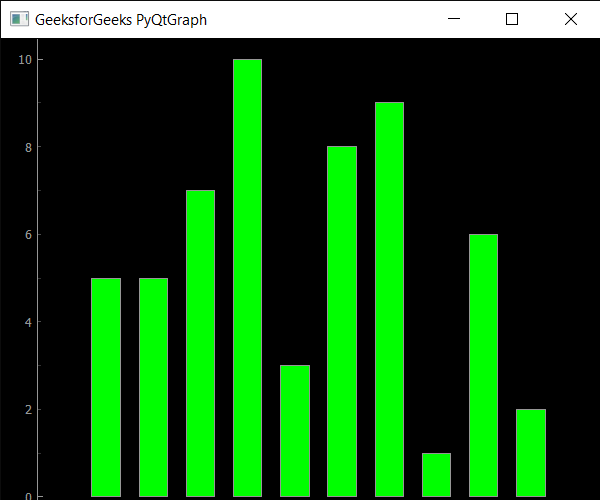Related Articles
PyQtGraph – Getting Maximum Size of Plot Window
• Last Updated : 25 Sep, 2020

In this article we will see how we can get maximum size of the plot window in the PyQtGraph module. PyQtGraph is a graphics and user interface library for Python that provides functionality commonly required in designing and science applications. Its primary goals are to provide fast, interactive graphics for displaying data (plots, video, etc.) and second is to provide tools to aid in rapid application development (for example, property trees such as used in Qt Designer).Plot windows consist of two main parts: the Plot Panel containing the actual plotted graphics and the Control Panel. By default plot window can be resized, by setting maximum size we assure that user will not be able to resize the window above the maximum size point. It can be set with the help of `setMaximumSize` method.

We can create a plot window with the help of command given below

```# creating a pyqtgraph plot window
window = pg.plot()
```

In order to do this we use `maximumSize` method with the plot window object

Syntax : window.maximumSize()

Argument : It takes no argument

Return : It returns QSize object

Below is the implementation

 `# importing pyqtgraph as pg``import` `pyqtgraph as pg`` ` `# importing QtCore and QtGui from the pyqtgraph module``from` `pyqtgraph.Qt ``import` `QtCore, QtGui`` ` `# importing numpy as np``import` `numpy as np`` ` `import` `time`` ` `# creating a pyqtgraph plot window``window ``=` `pg.plot()`` ` `# setting window geometry``# left = 100, top = 100``# width = 600, height = 500``window.setGeometry(``100``, ``100``, ``600``, ``500``)`` ` `# title for the plot window``title ``=` `"GeeksforGeeks PyQtGraph"`` ` `# setting window title to plot window``window.setWindowTitle(title)`` ` `# create list for y-axis``y1 ``=` `[``5``, ``5``, ``7``, ``10``, ``3``, ``8``, ``9``, ``1``, ``6``, ``2``]`` ` `# create horizontal list i.e x-axis``x ``=` `[``1``, ``2``, ``3``, ``4``, ``5``, ``6``, ``7``, ``8``, ``9``, ``10``]`` ` `# create pyqt5graph bar graph item ``# with width = 0.6``# with bar colors = green``bargraph1 ``=` `pg.BarGraphItem(x ``=` `x, height ``=` `y1, width ``=` `0.6``, brush ``=``'g'``)`` ` `# add item to plot window``# adding bargraph item to the window``window.addItem(bargraph1)`` ` `# setting maximum size of plot window``window.setMaximumSize(``600``, ``500``)`` ` `# getting maximum plot window size``value ``=` `window.maximumSize()`` ` `# printing the value``print``(``"Maximum Size : "` `+` `str``(value))`` ` ` ` ` ` `# main method``if` `__name__ ``=``=` `'__main__'``:``     ` `    ``# importing system``    ``import` `sys``     ` `    ``# Start Qt event loop unless running in interactive mode or using ``    ``if` `(sys.flags.interactive !``=` `1``) ``or` `not` `hasattr``(QtCore, ``'PYQT_VERSION'``):``        ``QtGui.QApplication.instance().exec_()``        `

Output :```Maximum Size : PyQt5.QtCore.QSize(600, 500)
```

Attention geek! Strengthen your foundations with the Python Programming Foundation Course and learn the basics.

To begin with, your interview preparations Enhance your Data Structures concepts with the Python DS Course. And to begin with your Machine Learning Journey, join the Machine Learning – Basic Level Course

My Personal Notes arrow_drop_up